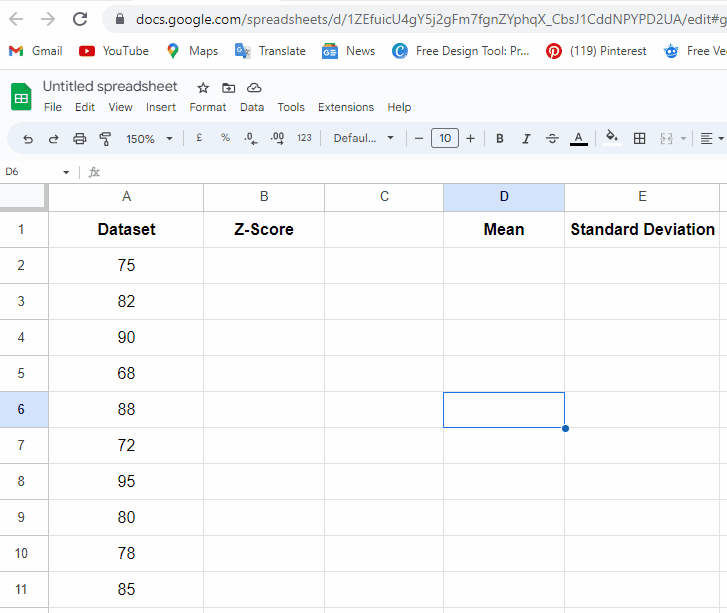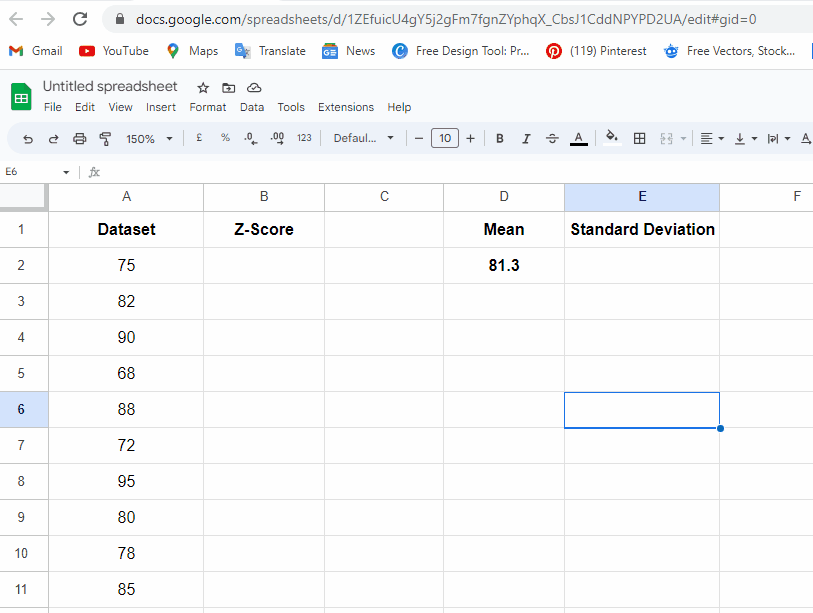# How To Calculate Z Score In Google Sheets

The z-score, also known as the standard score, is a statistical measure that helps us assess how far a particular data point deviates from the mean of a dataset, expressed in terms of standard deviations. It provides a standardized way of comparing and evaluating data points from different distributions. By calculating z-scores, we can determine whether a value is relatively high or low within its dataset and identify outliers or extreme observations.

The formula to calculate the z-score of a data point is straightforward. It involves subtracting the mean of the dataset from the data point and then dividing the result by the standard deviation of the dataset. Mathematically, it can be expressed as:

z = (x – μ) / σ

Where,

• z represents the z-score
• x is the individual data value
• μ is the mean of the dataset
• σ denotes the standard deviation

Let’s understand it with the following dataset.When it comes to statistical analysis and data interpretation, calculating z-scores plays a vital role in understanding the relative position of data points within a distribution. Whether you’re analyzing exam scores, financial data, or any other dataset, knowing how to calculate z-scores in Google Sheets can provide valuable insights. In this article, we will explore the concept of z-scores, their significance, and guide you through the process of calculating z-scores using the built-in functions in Google Sheets.

### Step 1 – Calculate Mean (Average)– First, we will calculate the Mean (Average).
– Go to cell D2 & type the formula: =AVERAGE(A2:A11) to calculate the mean.

### Step 2 – Calculate Standard Deviation– Now, go to cell E2 to calculate the value of the standard deviation.
– Type formula: =STDEV.P(A2:A11).
– Press the enter button.

### Step 3 – Calculate ZSCORE– Now we will calculate the ZSCORE.
– Go to cell B2 & type the formula: =(A2-\$D\$2)/\$E\$2).
* Press the enter button.